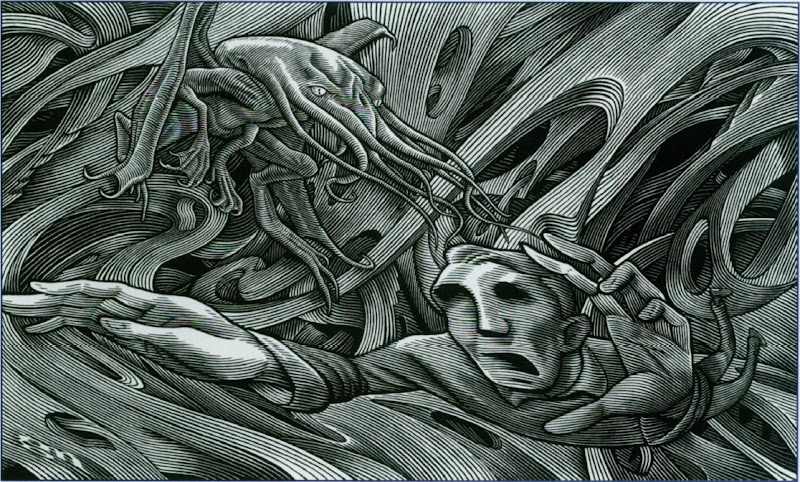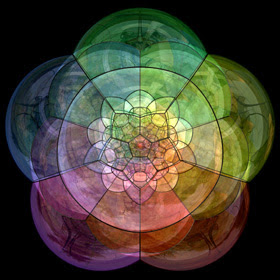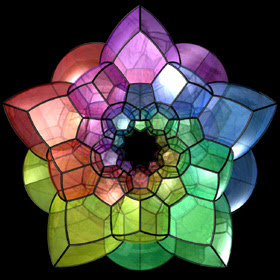### Lovecraft's mathematical horrors

Sometimes we can find on the web something of interesting, like the following review of the 4D Man.
Brilliant but irresponsible scientist Tony Nelson (James Congdon) develops an amplifier that allows any object to achieve a 4th dimensional (4D) state. While in this state that object can pass freely through any other object.
Reading these words I immediatly think to Howard Philips Lovecraft and his Cthulhu Mythos, in particular to Dream in the Witch House. In this short story Walter Gilman, a student of mathematics, lives in the house of Keziah Mason, one of the Salem's witches. In the story there are some mathematically interesting quotes:
She had told Judge Hathorne of lines and curves that could be made to point out directions leading through the walls of space to other spaces beyond (...)
We can argue the Lovecraft's use for his purpouse of the non-euclidean geometry, in particular in the following quotation:
[Gilman] wanted to be in the building where some circumstance had more or less suddenly given a mediocre old woman of the Seventeenth Century an insight into mathematical depths perhaps beyond the utmost modern delvings of Planck, Heisenberg, Einstein, and de Sitter.
or in the following point, in which HPL seems refer to Riemann's hypotesys:
He was getting an intuitive knack for solving Riemannian equations, and astonished Professor Upham by his comprehension of fourth-dimensional and other problems (...)
Indeed Gilman was studying
non-Euclidean calculus and quantum physicsIllustration by Greg Nemec
And Walter, dreaming, has experienced the high dimensional space of the limitless abysses:
abysses whose material and gravitational properties, and whose relation to his own entity, he could not even begin to explain. He did not walk or climb, fly or swim, crawl or wriggle; yet always experienced a mode of motion partly voluntary and partly involuntary. Of his own condition he could not well judge, for sight of his arms, legs, and torso seemed always cut off by some odd disarrangement of perspective; (...)
Durign his travel in the fourth-dimension, Gilman seen
risms, labyrinths, clusters of cubes and planes, and Cyclopean buildings
that are characteristic in lovecraftian literature.
Another non-euclidean reference is in The Call of Cthulhu(1):
He said that the geometry of the dream-place he saw was abnormal, non-Euclidean, and loathsomely redolent of spheres and dimensions apart from ours.
And Cthulhu itself is a fourth dimensional creature. Cthulhu was one of the Great Old Ones: these creatures
(...) were not composed altogether of flesh and blood. They had shape (...) but that shape was not made of matter.
We can imagine Cthulhu in our world like the projection of a dodecaplex in a three dimensional space, for example:The images was realized by Paul Nylander using Pov-Ray
Some other interesting figure come from projection of 4-dimensional solids are the 4-dimensional spheres in our 3-dimensional geometry:Claudio Rocchini on Commons
The hyperspheres(2) have a most important role in mathematics and topology. Indeed in 1900 Henri Poincaré stated his famous conjecture:
Every simply connected, closed 3-manifold is homeomorphic to the 3-sphere.
But... what is a manifold?
This mathematical object was introduced by Riemann in 1851 in his doctoral thesis: he had need to introduce in his work some highdimensional objects, thereby affecting the non-Euclidean geometries. The manifolds, indeed, are particular spaces in which Euclidean geometry is valid only in small portions, but loses its validity as we study more great portions of the manifold. A three-dimensional manifold is the sphere, for example: if we drawn a triangle on a sphere, the sum of its interior angles is 230°, while on the plane the sum is 180°. So while the surface of the sphere as a whole is governed by a non-Euclidean geometry, a small portion of his surface has the Euclidean properties: you could then simply say that a manifold is a space whose geometrical properties vary according to the watching scale.
A simply connected space is an indivisible space, meaning that it cannot be divided into other subspaces, both closed or open (and this is the connection) and, above all, every closed curve can be deformed to coincide with a point (and then the connected space becomes simply connected). Example of a simply connected space is, once again, a sphere.
In order to explain closure, I make an example. Let us consider a metric space $X$, a space where you can define a distance $d$ between two given points $x$, $y$. Then let us consider a subspace $M$ of $X$: we can define the distance of any point $a$ from space $M$ as the minimum distance between $a$ point and $x$ of $M$. The closure of $M$ will consist of all points of $X$ whose minimum distance of $M$ is zero. Surely all points of $M$ belong to the closure of of $M$ (each point is in fact at distance zero from himself), which must be added that can be defined as the edge of $M$. Then a subspace can be defined as closed if it coincides with its closure.
And finally the homeomorphism.
First of all we must define the application (or map): it is a function that associates to every point $x$ the value $f(x)$. An application $f$ is an omeomorphism if it is one-to-one (to every $x$ there is one and only one $f (x)$) and if it preserves the closure. In other words if the transformation of the closure of the space $M$ coincide with the closure of the transformation of the space $M$: $f(\overline{M})=\overline{f(M)}$ So it could be more clear the translation of the conjecture:
It exists a way to transform every geometrical shapes close and without hole in a 4th dimensional space in a 4th dimensional sphere.
The conjecture became a real mathematical theorem in 2010 when Clay Institute accepted Perelman's demonstration(4, 5, 6).
I have had many severecriticism because of the concrete and tangible nature of some of my "cosmic horrors". Variants of the general theme include defeats of the visible laws of time (...) and transcensions of the boundary-lines of Euclidean space.
(HP Lovecraft)

An interesting article about Lovecraft and Mathematics is H.P. Lovecraft: a Horror in Higher dimensions by Thomas Hull. Nemec's illustration comes from this paper.
Sagan's video from Miskatonic Museum
(1) In this short story there is also another mathematical reference:
After vigintillions of years great Cthulhu was loose again, and ravening for delight.
where vigintillions is 1063
(2) Exotic spheres, or why 4-dimensional space is a crazy place by Richard Elwes
(4) The entropy formula for the Ricci flow and its geometric applications
(5) Ricci flow with surgery on three-manifolds
(6) Finite extinction time for the solutions to the Ricci flow on certain three-manifolds